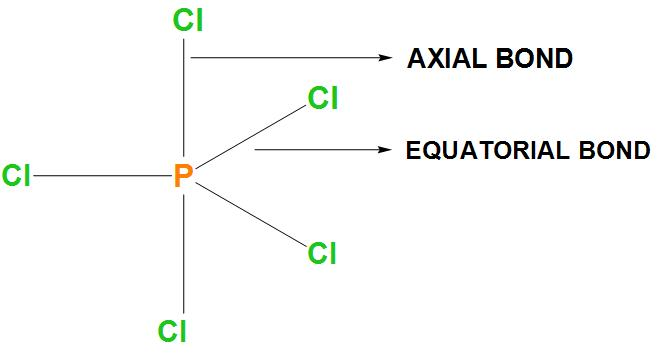QuestionAnswers

# P in $PC{{l}_{5}}$ has $s{{p}^{3}}d$ hybridization which of the following statements is wrong about structure?A. two P-Cl bonds are stronger and three P-Cl bonds are weakerB. two P-Cl bonds are axial and larger than three P-Cl equatorial bonds.C. $PC{{l}_{5}}$has trigonal bipyramidal geometry with non-polar natureD. All of theseVerified
92.4k+ views
Hint: To solve this question, we should use the VSEPR theory, according to this theory we can find the shape from hybridisation. In $PC{{l}_{5}}$ there are two types of P-Cl bonds present, that are axial and equatorial. The longer bond is weaker and the shorter among them is the stronger bond.

- In the first option it is said that two P-Cl bonds are stronger and three P-Cl bonds are weaker. As we know that there are 3 equatorial bonds and 2 axial bonds and the axial bonds are basically longer. Let’s draw the structure of $PC{{l}_{5}}$:Hence, we can conclude that the correct option is (a), that is the statement which is wrong about structure of $PC{{l}_{5}}$ is: two P-Cl bonds are stronger and three P-Cl bonds are weaker.
- It is found that $PC{{l}_{5}}$ is a chemical compound that is mainly used as a chlorinating reagent.it is found that $PC{{l}_{5}}$ converts alcohols to alkyl chlorides and carboxylic acids to acyl chlorides. But its structure depends on the environment, in the gaseous and molten state it has trigonal bipyramidal geometry and in solid state it becomes ionic compound, $PCl_{4}^{+},PCl_{6}^{-}$